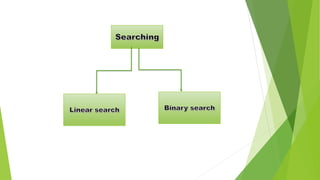Seu SlideShare está sendo baixado. ×

# Searching and sorting

Anúncio
Anúncio
Anúncio
Anúncio
Anúncio
Anúncio
Anúncio
Anúncio
Anúncio
Anúncio
AnúncioCarregando em…3
×

1 de 20 Anúncio

# Searching and sorting

Searching and sorting
Types of Searching
1. Linear Searching
2. Binary Searching
Types of Sorting
1.Selection Sort
2. Insertion Sort
3.Bubble Sort
And the examples of Linear searching, Binary Searching
And also the examples of Selection sort, Insertion sort and Bubble sort and describing them in detail in this ppt

Searching and sorting
Types of Searching
1. Linear Searching
2. Binary Searching
Types of Sorting
1.Selection Sort
2. Insertion Sort
3.Bubble Sort
And the examples of Linear searching, Binary Searching
And also the examples of Selection sort, Insertion sort and Bubble sort and describing them in detail in this ppt

Anúncio
Anúncio

### Searching and sorting

1. 1. SEARCHING AND SORTING
2. 2. SEARCHING Searching is a method of finding a element position from group of elements in data structure, such as array, linked list, tree or graph.
3. 3. LINEAR SEARCH :  Linear search is also called as sequential search.  It is a basic and simple search algorithm.  Linear search starts at the beginning of the list and checks every element in the list.  Linear search compares the element with all the other elements given in the list. If the element is matched it returns the valve index, else it returns -1.
4. 4. HOW LINEAR SEARCH WORKS ? Now, let's see the working of the linear search Algorithm. let's take an unsorted array. It will be easy to understand the working of linear search with an example. Let the elements of array are - Let the element to be searched is K = 41 Now, start from the first element and compare K with each element of the array. The value of K, i.e., 41, is not matched with the first element of the array. So, move to the next element. And follow the same process until the respective element is found.
5. 5. Now, the element to be searched is found. So algorithm will return the index of the element matched.
6. 6. BINARY SEARCH :  Binary search is used for searching an element in a sorted array.  It is useful when there are large number of element in an array.  These searching technique looks for a particular element by comparing the middle most element of the collection.
7. 7. How does Binary search work ? Now, let’s see the working of the Binary search algorithm. Let the elements of array are- Let the element to be searched is, K=56
8. 8. Now, the element to search is found. So algorithm will return the index of the element matched.
9. 9. SORTING : Sorting is a method of elements of array in a particular format either ascending or descending.
10. 10. Insertion sort Selection sort Bubble sort Sorting
11. 11. INSERTION SORTING :  Insertion sort algorithm arranges a list of elements.  In Insertion sort algorithm every iteration moves an element from unsorted to sorted portion.  Until all the elements are sorted in the list.  This algorithm is not suitable for the large data set.
12. 12. How does Insertion sort work ?
13. 13. SELECTION SORTING :  In Selection sorting algorithm the first element in the list is selected and it is compared with all the remaining elements in the list.  If any element is smaller than the selected element then the both are swapped.  So, the first position is filled with smallest element.  This procedure is repeated until the entire list is sorted.
14. 14. How does Selection sort work ? Now, let's see the working of the Selection sort Algorithm. To understand the working of the Selection sort algorithm, let's take an unsorted array. Let the elements of array are –
15. 15. Now, the array is completely sorted.
16. 16. BUBBLE SORTING :  Bubble sort is the simplest sorting algorithm . It always arrange the data in descending order.  Bubble sort algorithm is not suitable for the huge data set.  Bubble sort algorithm compares two items at a time and swapping them if they are in the wrong order.  The iteration is repeated until no swaps are needed, which means list is sorted.
17. 17. How does Bubble sort work ? Now, let’s see the working of the Bubble sort Algorithm. Suppose we have a list of array of 5 elements A={40,50,30,20,10} Iteration-I: A=40 40 40 40 40 40 A=50 50 50 30 30 30 A=30 30 30 50 20 20 A=20 20 20 20 50 10 A=10 10 10 10 10 50
18. 18. Iteration-II: 40 30 30 30 30 40 20 20 20 20 40 10 10 10 10 40 50 50 50 50 Iteration-III: 30 20 20 20 30 10 10 10 30 40 40 40 50 50 50
19. 19. Iteration-IV: 20 10 10 10 20 20 30 30 30 40 40 40 50 50 50 Presented by: Ch.Sreeja Ch.Sandeep K.Bhavani S.Anil R.Mounika